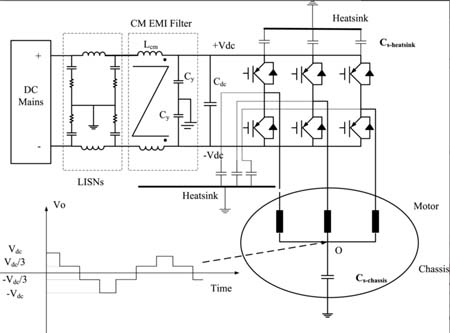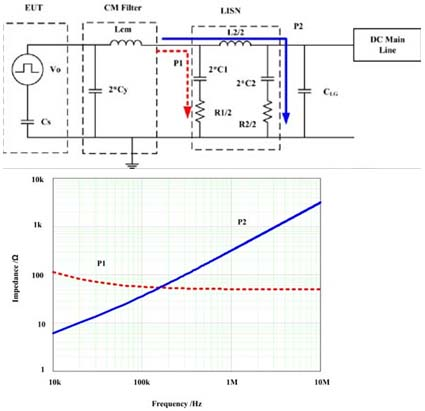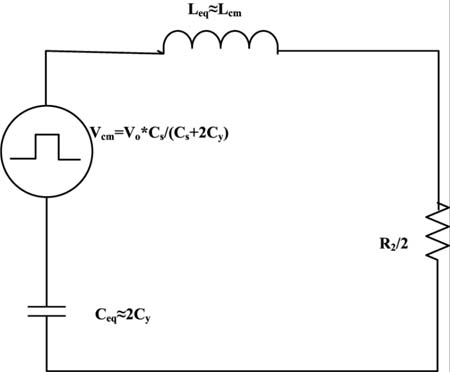LIBRARY

# Analysis of CM Volt-Second Influence on CM Inductor Saturation and Design for Input EMI Filters in Three Phase DC-Fed Motor Drive

Year: 2010Fig. 1 . Motor drive EMI test system
The saturation of the CM inductor core is a complex and important issue in EMI (Electro Magnetic Interference) filter design. In previous literatures, it has been proven that saturation can be caused by the leakage inductance. But in practice, the common-mode choke can still be saturated even if it's designed with leakage inductance and DM current constraints. Although overdesigning the magnetic component is a safe way to prevent the core from saturation, the resulting bulky size is not desirable for high-power-density converter design.

In this paper, a new mechanism of CM choke saturation due to CM voltage in motor drive system is investigated, in additional to previous analysis on CM choke saturation. Fig.1 shows the motor drive system configuration. In this system the neutral point CM voltage can be considered as the noise source, which determines the CM volt-second of CM inductor. Here LISNs play a significant role in the CM noise propagation. Fig. 2 shows the input impedance of LISN paths. For low frequencies, LISN impedance is less than 50 ohm. Hence LISN creates a low impedance path to ground. Motor drive switching frequency is at tens of kHz in most applications, so the CM equivalent circuit of motor drive system can be determine by Figure.3.The CM equivalent circuit can be simplified to be a RLC resonant circuit and the CM volt-second on CM inductor can be quantified. The CM inductor volt-second is determined by both CM propagation path parameters, modulation scheme and operating point. With the calculated volt-second, in additional to leakage inductance, the minimum volume of CM choke can be designed.Fig. 2. CM equivalent circuitFig. 3. Simplified CM equivalent circuit for CM volt-second calculation